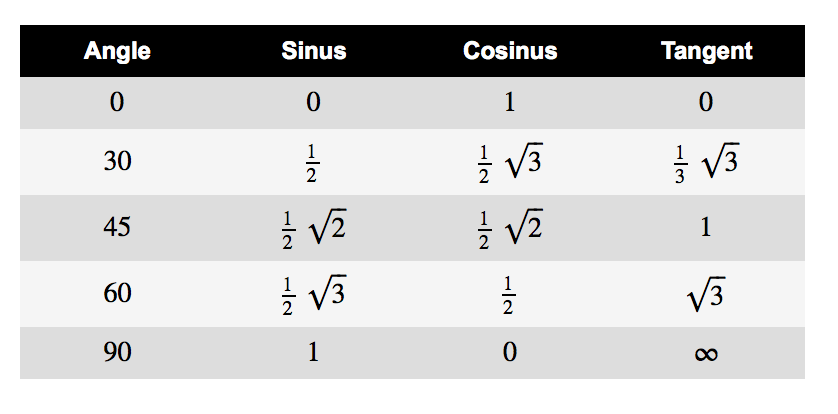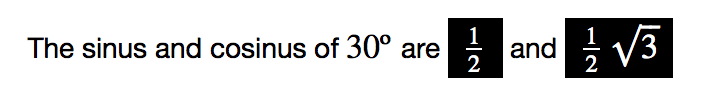# color in latex...

1) Is it possible to use text colors in show specifically with LatexExpr.

2) in mathematica, it is possible to insert a result in a text cell. Is it possible in SM markdown ?

3) same question in an HTML table ? SM tables are too basic

4) Need an exemple to localy change the background color of a result.

edit retag close merge delete

"SM"=Sagemath, I suppose ? This abbreviation is a tad ambiguous (unless you really want to suggest that Sagemath==SM=sadomasochism ? This would be questioned by some...).

I have had also this idea but I was wondering that I was alone to have a bad spirit. I was using SM as MA for Mathematica. However it seems we need an acronym for Sagemath.

Sort by » oldest newest most voted1) Yes in a Jupyter notebook. See an example below.

2) You have already asked something similar in this other question. I think that the answers there also apply here.

3) Certainly you can put results inside HTML tables generated by code cells. Example:

Degrees = pi/180
angles = [0, 30, 45, 60, 90]
st = "style='text-align: center'"
tb = f"""
<table style='width:50%'>
<tr style='color: white; background: black'>
<th {st}>Angle</th>
<th {st}>Sinus</th>
<th {st}>Cosinus</th>
<th {st}>Tangent</th>
</tr>
"""
for a in angles:
tb += f"<tr><td {st}>${latex(a)}$</td>"
tb += f"<td {st}>${latex(sin(a*Degrees))}$</td>"
tb += f"<td {st}>${latex(cos(a*Degrees))}$</td>"
tb += f"<td {st}>${latex(tan(a*Degrees))}$</td></tr>"
tb += "</table>"
show(html(tb))


To understand the code, google for Python f-string. I provide a screen capture of the result:4) Voici un exemple:

Degrees = pi/180
angle = 30
st = "style='background: black; color: white; padding: 0.5em'"
message = fr"The sinus and cosinus of ${latex(angle)}º$ are "
message += fr"<span {st}>${latex(sin(angle*Degrees))}$</span> and "
message += fr"<span {st}>${latex(cos(angle*Degrees))}$</span>"
show(html(message))


And the corresponding screen capture:As you can see, I don't use LaTeXExpr, but latex. Likewise, formulae are coloured as a whole via CSS. If you need to change the color of only a part of an expression, then you should use the \color macro.

more

1) Yes in the (Jupyter) notebook (Mathjax happens to understand \color...) . No in command-line (or emacs), unless you add the relevant \usepackage{xcolor} or analogous to the relevant latex preamble (see the docs).

2) Not as far as know. This is much better achieved in a SageTeX (or org-mode + sage-shell-mode in emacs) document (with the right modules in the preamble). Again, see the SageTeX documentation and example.

3) This might be doable by writing Sage code to spit out HTML results. ISTR that Sage has some relevant functions for that, but never used it. See the docs, again. An alternative is org-mode with HTML output.

4) is highly dependent of your output mode. There might be some Methjax happenstance, I dunno. See the relevant docs.

sage: r('library("fortunes")')
 "fortunes"  "tools"     "stats"     "graphics"  "grDevices" "utils"
 "datasets"  "methods"   "base"
sage: r('fortune("RTFM")')

This is all documented in TFM. Those who WTFM don't want to have to WTFM again
on the mailing list. RTFM.
-- Barry Rowlingson
R-help (October 2003)

more

the syntax of the Latex \color statement (not quite obvious):

show(LatexExpr( r" {\color{Red} {tagada}}"))